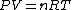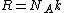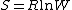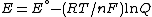# gas constant

(redirected from Molar gas constant)
Also found in: Dictionary, Thesaurus, Medical.

## Gas constant

The universal constant R

(1)that appears in the ideal gas law, Eq. (1), where P is the pressure, V the volume, n the amount of substance, and T the thermodynamic (absolute) temperature. The gas constant is universal in that it applies to all gases, providing they are behaving ideally (in the limit of zero pressure). The gas constant is related to the more fundamental Boltzmann constant, k, by
(2)Eq. (2), where NA is the Avogadro constant (the number of entities per mole). The best modern value in SI units is R = 8.314 472 (15) J/K &cdot; mol, where the number in parentheses represents the uncertainty in the last two digits. See Boltzmann constant

According to the equipartition principle, at a temperature T, the average molar energy of each quadratic term in the expression for the energy is (1/2)RT; as a consequence, the translational contribution to the molar heat capacity of a gas at constant volume is (3/2)R; the rotational contribution of a linear molecule is R. See Kinetic theory of matter

Largely because R is related to the Boltzmann constant, it appears in a wide variety of contexts, including properties unrelated to gases. Thus, it occurs in Boltzmann's formula for the molar entropy of any substance, Eq. (3),

(3)where W is the number of arrangements of the system that are consistent with the same energy; and in the Nernst equation for the potential of an electrochemical cell, Eq. (4),
(4)where E° is a standard potential, F is the Faraday constant, and Q is a function of the composition of the cell. The gas constant also appears in the Boltzmann distribution for the population of energy levels when the energy of a level is expressed as a molar quantity. See Boltzmann statistics, Entropy

McGraw-Hill Concise Encyclopedia of Physics. © 2002 by The McGraw-Hill Companies, Inc.

## gas constant

[′gas ‚kän·stənt]
(thermodynamics)
The constant of proportionality appearing in the equation of state of an ideal gas, equal to the pressure of the gas times its molar volume divided by its temperature. Also known as gas-law constant; universal gas constant.
McGraw-Hill Dictionary of Scientific & Technical Terms, 6E, Copyright © 2003 by The McGraw-Hill Companies, Inc.
References in periodicals archive ?
where [[bar.R].sup.Lem] is the universal molar gas constant used by Lemmon et al.
where [[bar.R].sup.97] is the molar gas constant of IAPWS-IF97, given in Table 3, [pi] is the reduced pressure, and [tau] is the reciprocal reduced temperature.
where [[bar.R].sup.95] is the molar gas constant of IAPWS-95, given in Table 2, [tau] is the reciprocal reduced temperature, and [delta] is the reduced density.
where [[psi].sub.w] is the mole fraction of water vapor, [[bar.s].sub.a.sup.o] is the ideal-gas molar entropy of dry air [[bar.s].sub.w.sup.o] is the ideal-gas molar entropy of water vapor--both described below, [bar.v] is the molar volume of moist air calculated iteratively using Equation 47, [B.sub.m] and [C.sub.m] are calculated using Equations 10 and 11, d[B.sub.m]/dT and d[C.sub.m]/dT are calculated using Equations 12 and 13, and [bar.R] is the universal molar gas constant, given in Table 5.
where [[bar.R].sup.Lem] is the universal molar gas constant used by Lemmon et al., given in Table 1, [tau] is the reciprocal reduced temperature, [delta] is the reduced density, [[alpha].sup.o] is the ideal-gas part of the reduced fundamental Lemmon et al.

Site: Follow: Share:
Open / Close